Affiliated Institutes

### The MS in Computational & Applied Mathematics trains you in the analytical and numerical methods of applied mathematics to address and solve pressing challenges in all areas of science and engineering.

Mathematical modeling and scientific computing have become increasingly useful problem-solving tools. You’ll learn analytical and numerical methods of applied mathematics, applying them to problems in physics, biology, engineering, economics, climate science, and more. You’ll work alongside faculty who have extensive backgrounds in applied mathematics, computational science, statistics, financial engineering, industrial modeling, and related fields. Our program produces educated, capable practitioners who excel at communicating knowledge and solving complex mathematical challenges across disciplines. Join a new generation of mathematical scientists ready for leadership roles in education, industry, government laboratories, technology companies, and more.

## Program At a Glance

UNITS
32 units

COMPLETION TIME
2 years

COURSES BEGIN
Fall | Spring

DEGREE AWARDED
MS in Computational & Applied Mathematics

## Featured Courses

MATH 294
Methods of Applied Mathematics

Studies derivation of classical equations of applied math including quasilinear hyperbolic equations, Laplace, Poisson and Helmholtz equations, among others, in various orthogonal curvilinear coordinate systems.

MATH 368
Numerical Methods for Matrix Computations

Examines numerical linear algebra and the matrix computations that arise in solving linear systems, least-squares problems, and eigenvalue problems for both dense and sparse matrices.

MATH 362
Numerical Methods for Differential Equations

Studies numerical integration of ordinary and partial differential equations through methods such as Runge-Kutta and Adams formulas and more.

Math 462
Mathematics of Machine Learning

Covers theoretical foundations, algorithms, and methodologies for machine learning, emphasizing the role of probability and optimization and exploring a variety of real-world applications.

MATH 256
Stochastic Processes

Analyzes properties of independent and dependent random variables and examines conditional expectation among topics chosen from Markov processes and others.

MATH 306
Optimization

Emphasizes nonlinear programming and numerical methods for finite-dimensional optimization problems with fairly smooth functions.

Curriculum

Degree Requirements
A minimum of eight courses (32 units) of graduate math coursework is required, at least five of which (20 units) must be at the 300-level or higher.

Students who lack the prerequisite undergraduate coursework may be asked to complete more than 32 units. At least 20 units of coursework must be gamma courses (300-level and above). A grade of B- or above must be earned in gamma courses.

Students in the master’s program in mathematics, computational and applied mathematics, and statistical sciences may convert one 200-level (beta) course to gamma credit. At the discretion of the IMS Director, in consultation with the student’s academic advisor, more than one conversion may be approved in exceptional cases.

Core Courses (Four courses – 16 units)

• Methods of Applied Mathematics (waived if the student has had equivalent preparation: complex variables, vector calculus, Fourier series/transforms, and PDEs)
• Numerical Methods for Matrix Computations
• Numerical Methods for Differential Equations
• Continuous Mathematical Modeling, or Discrete Mathematical Modeling

Restricted Electives (Two electives – 8 units)

• Any of the core courses listed above if not taken to satisfy the core requirements
• Mathematics Clinic
• Stochastic Processes
• Optimization
• Reliability Theory
• Deterministic and Stochastic Control
• Mathematical Finance
• Simulation
• Fluid Dynamics
• Perturbation and Asymptotic Analysis
• Mathematical Modeling in Biology
• Image Processing

Independent Study
In lieu of one formal course, the student may take Math 398 Independent Study with a research advisor leading to a publication quality technical report in an area of computational or applied math.

Accelerated Degree Option

If you are an undergraduate student at the Claremont Colleges*, you can obtain a graduate degree on an accelerated track. Undergraduate students can begin working toward the master’s requirements simultaneously with the completion of their undergraduate degrees.

You can earn a Master’s Degree in Mathematics, Computational & Applied Mathematics, or Statistical Sciences from Claremont Graduate University with just one additional year. Up to 16 units of undergraduate credit may be accepted towards your graduate degree. Financial aid is available for your graduate year at CGU.

*The accelerated degree option is only open to undergraduate students at Pomona, Scripps, Claremont McKenna, Harvey Mudd, and Pitzer.

Consider this opportunity if:

• Your longer-term plans include a PhD in a math-related field (e.g. physics, engineering, computer science)
• You are seeking a teaching career in math or science
• You would like to get involved in ongoing research with CGU Math faculty during your senior year
• You would like to earn your Master’s degree in half the usual time

## Resources

Engineering & Computational Mathematics Clinic
CGU’s internationally recognized Engineering & Computational Mathematics Clinic offers first-hand experience solving significant mathematical problems for industry and government clients.

Recent projects include:

• Optimizing Transmission of Renewable Energy–Southern California Edison
• Hardware-Software Codesign–Los Alamos National Laboratory
• Data Cohort Analysis–Fair Isaac
• Optimizing Smart Power Grids–Los Alamos National Laboratory
• Credit Risk in a Network Economy–Fitch Rating
• Isogeometric Analysis–Boeing
• Gate to Base Capacitance Modeling for Nanoscale MOSFETs–USC Information Sciences Institute
• Practical Semi-Analytic Model for the Substrate Current of Short Channel MOSFETs with LDDs–USC Information Sciences Institute

Claremont Center for the Mathematical Sciences
Through the Claremont Center for the Mathematical Sciences (CCMS), you’ll have access to one of the largest mathematical science communities in California, as well as to workshops, conferences, and seminars, including:

• Southern California Analysis Seminar
• Math-in-Industry Workshop
• Michael E. Moody Lecture Series
• History and Philosophy of Mathematics Seminar
• Claremont Mathematics Weekend
• CCMS Software Lab
• and more

Faculty & Research
•John Angus

Professor of Mathematics

##### Research Interests

Probability, Statistics, Computing, Algorithms, Navigation, Systems Engineering, Mathematical Finance

•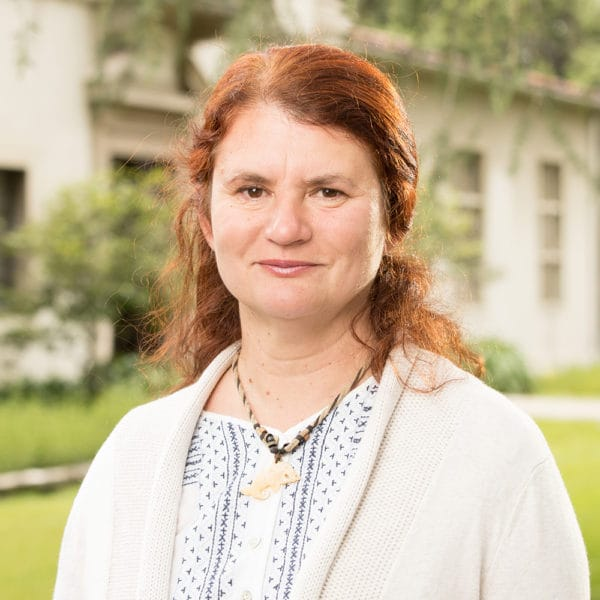Marina Chugunova

Professor of Mathematics
Program Director, PhD in Engineering & Computational Mathematics

##### Research Interests

Surfactant-driven thin film flows in biomedical applications; Nonlinear parabolic equations; Stability problems in fluid dynamics; Scientific computations; Applied operator theory; Sturm-Liouville problems

•Ellis Cumberbatch

Professor of Mathematics Emeritus

##### Research Interests

Applied mathematics, Industrial modeling, Differential equations, Fluid mechanics, Wave propagation, Semi-conductors

•Research Professor of Mathematics

##### Research Interests

Approximation theory, Computational harmonic analysis, Mathematics of data, Query processing

•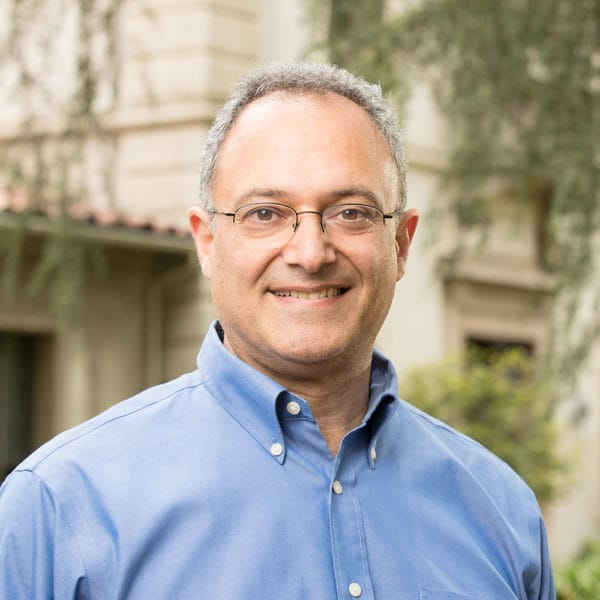Professor of Mathematics
Joseph H. Pengilly Chair in Mathematics

##### Research Interests

Fluid Dynamics, Mathematical Modeling, Scientific Computing

•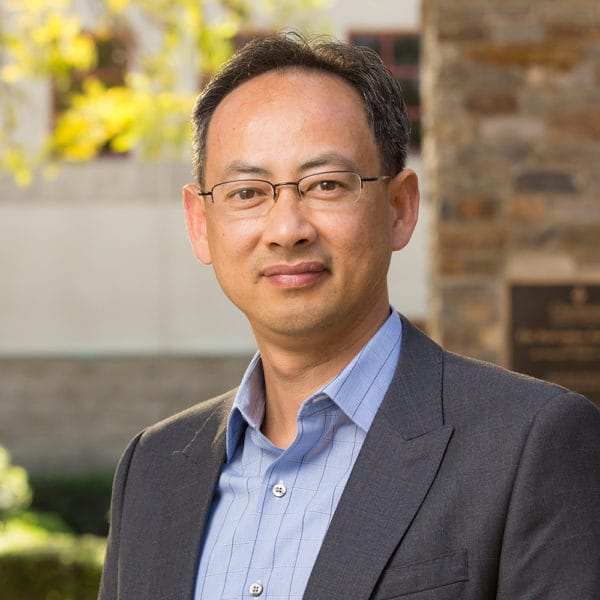Andrew Nguyen

##### Research Interests

Stochastic processes, Statistics, Risk management, Financial derivatives, Actuarial sciences, Statistical software

•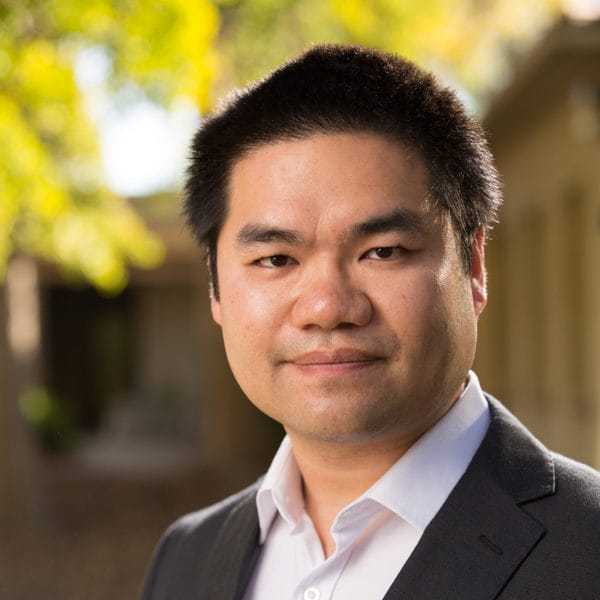Qidi Peng

Research Assistant Professor of Mathematics

##### Research Interests

Statistical inferences, Stochastic differential equations, Stochastic modeling, Simulation, Machine learning, Approximation theory, Graph theory

•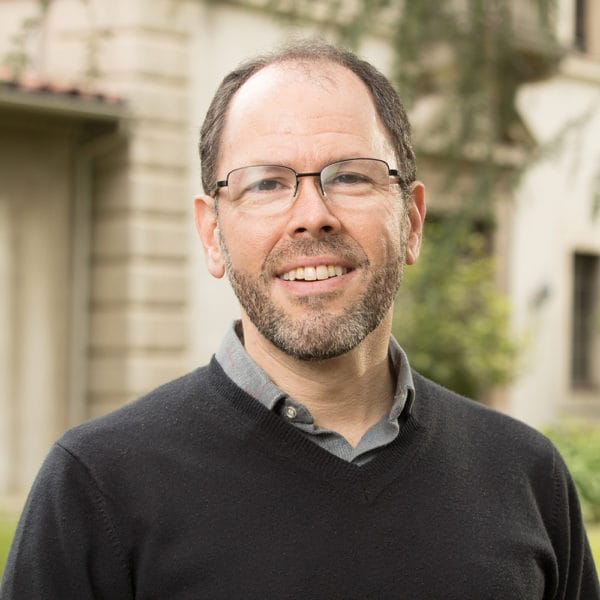Allon Percus

Professor of Mathematics

##### Research Interests

Discrete optimization; Network models; Statistical physics; Random combinatorial structures

•Claudia Rangel-Escareño

##### Research Interests

Probabilistic methods in computational biology, Statistical inference of genetic networks, Bioinformatics

•Henry Schellhorn

Professor of Mathematics
Director, Institute of Mathematical Sciences

##### Research Interests

Financial engineering, Credit risk, Stochastic analysis, Traffic models

## Extended Faculty

In addition to CGU core faculty, you will have access to Math faculty across the Claremont Colleges, including Pomona, Scripps, Harvey Mudd, Claremont McKenna, Pitzer, and Keck Graduate Institute, as well as faculty who are part of the W.M. Keck integrated science department.

Harvey Mudd
View Math Faculty

Pomona
View Math Faculty

Claremont McKenna
View Math Faculty

Scripps
View Math Faculty

Pitzer
David Bachman
Jim Hoste

W.M. Keck Integrated Science Department
(Claremont McKenna, Pitzer, Scripps)
John Milton

Animesh Ray
James Sterling

## Where You Can Find Our Alumni

• Raytheon

• RAND Corporation

• Memorial University of Newfoundland

• Stanford University

• Center for Infection and Immunity, Columbia University

• Jet Propulsion Laboratory

• ReResearch Nanotechnology

• Cablevision

• Accenture

### Request information about the Computational & Applied Mathematics program

• This field is for validation purposes and should be left unchanged.